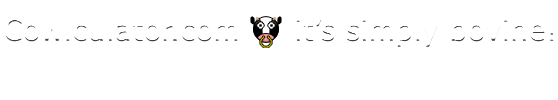Glossary - Symbols

One or more symbols are used to express mathematical formulae.  A partial list of symbols (currently being developed) is as follows:

 Symbols Meanings + positive, addition - negative, subtraction × mutliplication ÷ division * asterisk, multiplcation (computers) / slash, forward slash, division (computers) ⁄ fractional slash ± plus and minus (value can be of either sign) ° degree, angle ≡ identical to = equal sign < less than <= =< less than or equal to > greater than >= => greater than or equal to ≈ approximately equal to % per cent (n out of 100 parts) ‰ per mille (n out of 1,000 parts) ‱ per myriad (n out of 10,000 parts), per diem, basis point ¤ Currency (but not a specific currency) # octothorpe, number sign, cross-hatch ( ) opening and closing parenthesis (respectively), brackets ƒ florin, function π pi (the constant ratio of the circumference of a circle to its diameter) √ square root, radical sign ∞ infinity … horizontal ellipsis ′ imperial feet, minutes, [single] prime (not an apostrophe) ″ imperial inches, seconds, double prime (not a quotation mark)